# 计算问题

## 语言

$$L=\{\varepsilon, 0,1,10,11,100,110,111,\cdots\}$$

• 我们说”序列”就要考虑顺序, 即$001$和$100$是不同的串.
• 串可以是空串, 即长度为$0$的串, 空串通常记作$\varepsilon$.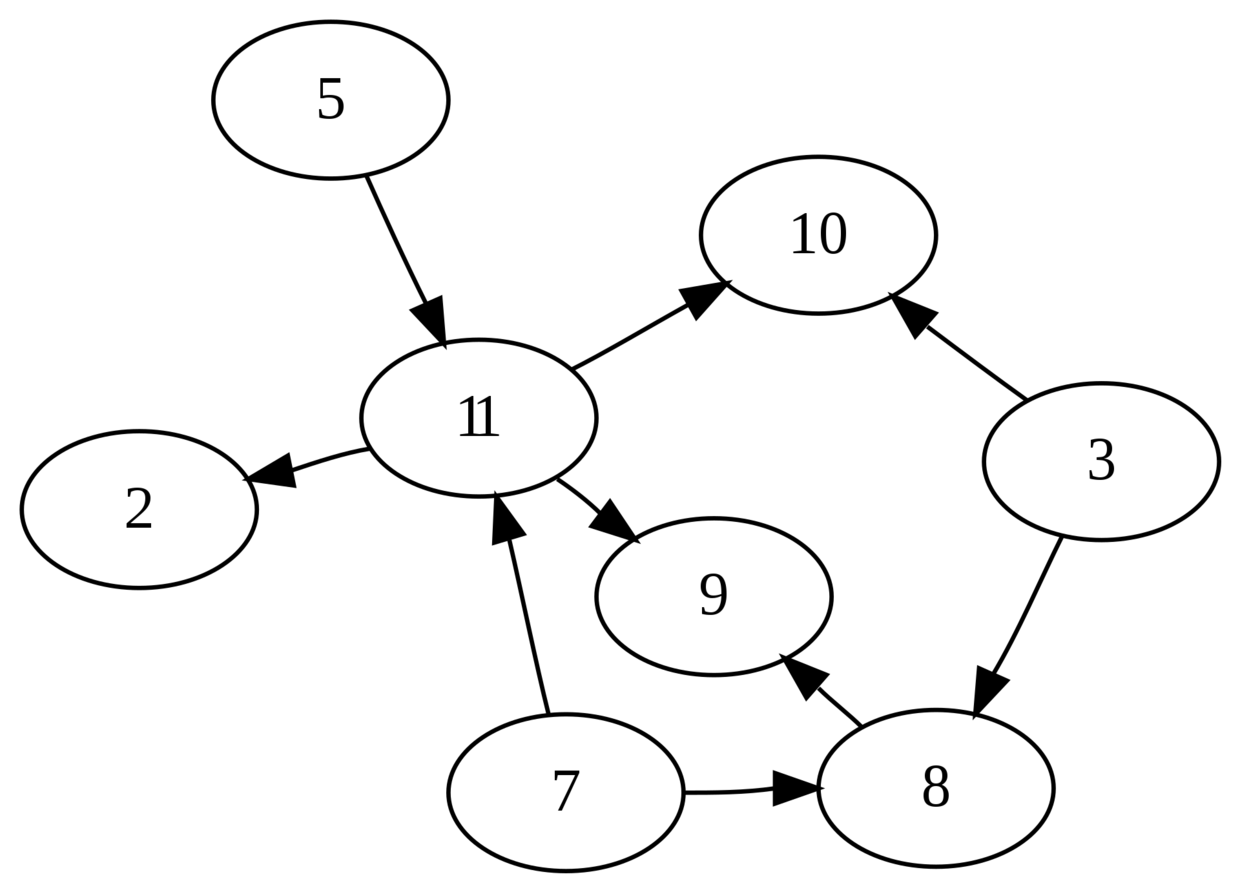$$100\dagger0010\cdot0011\cdot0101\cdot0111\cdot1000\cdot1001\cdot1010\cdot1010\cdot1011\dagger\\0011\to1000\cdot0011\to1010\cdot0011\to1010\cdot0101\to1011\cdot\\0111\to1000\cdot0111\to1011\cdot 1000\to1001\cdot1011\to0010\cdot\\10011\to1010\ddagger$$

$$0\to01,1\to10,\dagger\to00,\ddagger\to11,\cdot\to\varepsilon$$

# 自动机模型

## 有限状态机

1. $Q$是一个有限集, 称为状态集
2. $\Sigma$是一个有限集, 称为字母表
3. $\delta:Q\times\Sigma \to Q$称为转移函数
4. $q_0\in Q$是起始状态
5. $F\subseteq Q$是接受状态集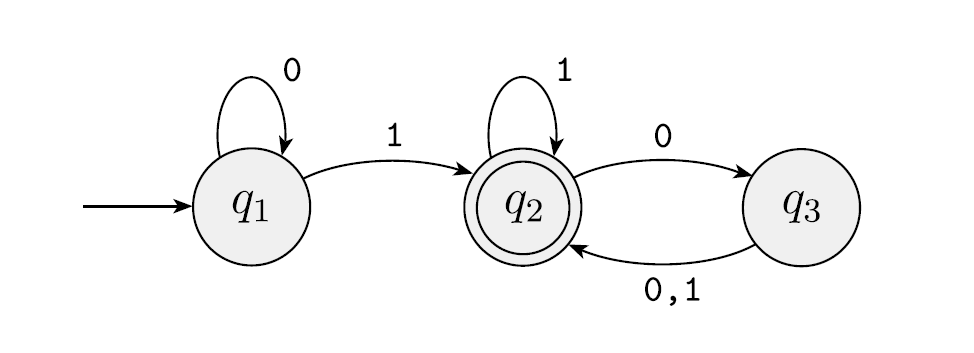• 圆圈表示状态

• 有一条没有起点的箭头指向的状态为起始状态
• 双圈表示的状态是接受状态
• 带符号集的箭头表示转移函数中的一组映射

例如$q_2\overset{0,1}{\longrightarrow}q_3$表示映射$(q_2,0)\mapsto q_3$和$(q_2,1)\mapsto q_3$两条映射

1. 有限状态机(finite state machine)也可以称为自动机(automaton), 但不能称为”有限自动机”.
2. 我们说”自动机”就是指”有限状态机”, 而不是”有限状态机””下推自动机””非确定自动机”等一系列计算模型的统称.

## 复杂度类REG

$$\textsf{REG}=\{L|\exists\text{自动机}M: x\in L\iff M(x)\}$$
REG表示那些能够被一台自动机识别的语言.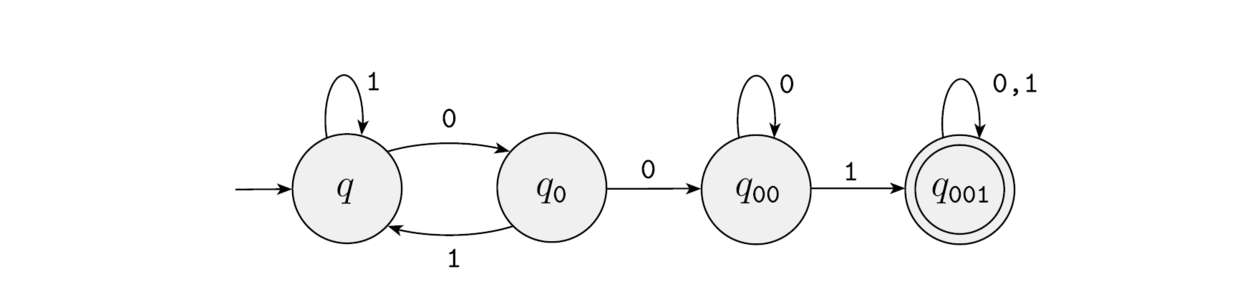# 正则语言与自动机

## 正则语言

$$L_1\circ L_2 = \{x_1x_2|x_1\in L_1, x_2\in L_2\}$$

$$L_1\cup L_2 = \{x|x\in L_1 或 x\in L_2\}$$

$$L^0=\{\varepsilon\}, \quad L^n=\{x^n|x\in L\} \\ L^\ast= \bigcup_{i\in\mathbb{N}} L^i$$

1. $L=\{a\}$, 其中$a$是某个字母表$\Sigma$上的符号;
2. $L=\{\varepsilon\}$;
3. $L=\emptyset$;
4. $L=L_1\cup L_2$, 其中$L_1$和$L_2$均为正则语言;
5. $L=L_1\circ L_2$, 其中$L_1$和$L_2$均为正则语言;
6. $L=L_1^\ast$, 其中$L_1$为正则语言;

1. 空集与任何语言作$\circ$或$\cup$运算得到的都是空集
2. $\emptyset^\ast=\{\varepsilon\}$

## 正则表达式

$$L=\{\varepsilon, 00, 01, 0000, 0100, 0101, \cdots\}$$

$$L= (L_0\circ (L_0\cup L_1))^\ast$$

1. $a$, 其中$a$是字母表$\Sigma$中的一个元素;
2. $\varepsilon$;
3. $\emptyset$;
4. $(R_1\cup R_2)$, 其中$R_1$和$R_2$是正则表达式
5. $(R_1\circ R_2)$, 其中$R_1$和$R_2$是正则表达式
6. $(R_1^\ast)$, 其中$R_1$是正则表达式

$$R= (10 | 01)  1$$

$$L={101,011,10001,01001}$$

1. $0^\ast 10^\ast=\{w|w恰好有一个1\}$
2. $(\Sigma\Sigma\Sigma)^\ast=\{w|w的长度正好是3的倍数\}$
3. $2333(3^\ast)=\{w|w是由2333开头且在之后是任意长度的纯3串\}$

## 非确定自动机

$$\emptyset,\{a\},\{b\},\{c\},\{ab\},\{ac\},\{bc\},\{abc\}$$

1. $Q$是一个有限集, 称为状态集
2. $\Sigma_{\varepsilon}$是一个有限集, 是字母表和空串$\varepsilon$的并集.
3. $\delta:Q\times\Sigma_\varepsilon \to \mathcal{P}(Q)$称为转移函数
4. $q_0\in Q$是起始状态
5. $F\subseteq Q$是接受状态集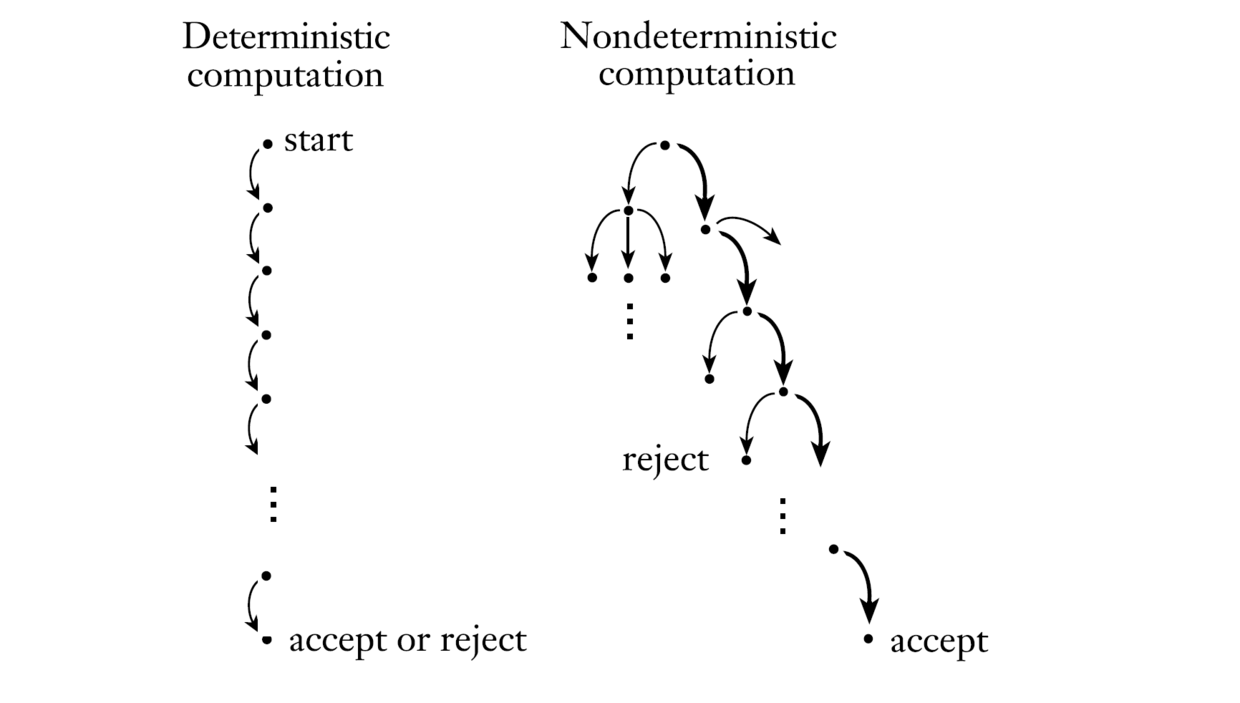1. 首先在起始状态时, 可能随机转移到状态$q_3$, 因此此时的合法状态集是$\{q_1,q_3\}$
2. 在收到第一个输入$b$时, 状态$q_1$只能转移到$q_2$, 而状态$q_3$根据输入$b$无法转移, 因此这一步的合法状态集为$\{q_2\}$
3. 在收到第二个输入$c$时, 状态$q_2$无法转移, 因此这一步的合法状态集为$\emptyset$.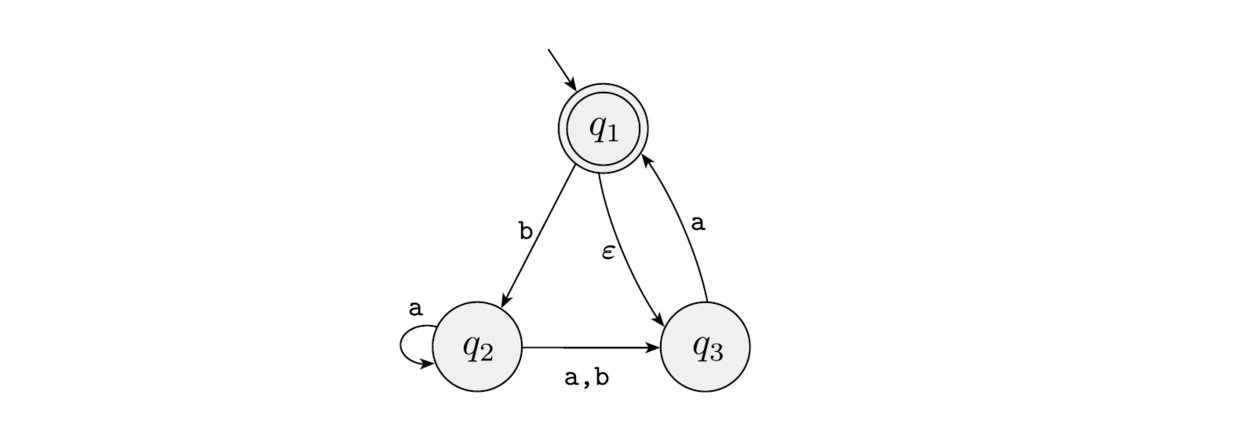## 非确定自动机的计算能力

1. 由于每一步计算都视为合法状态集到合法状态集的转换, 因此, $M$中的状态集应该是$Q$的超集, 即$\mathcal{P}(Q)$.
2. 由于自动机不能发生随机转移, 因此, $\varepsilon$不能参与转移, 即自动机的第二个参数退化为$\Sigma$.
3. 转移函数$\delta^\prime$肯定是一个$\mathcal{P}(Q)\times \Sigma \to \mathcal{P}(Q)$的集合, 显然这个转移是确定的, 因为每一个$\mathcal{P}(Q)$中的元素都能够被$M$的一个状态表示.
4. $M$的起始$q_0^\prime$状态是$q_0$及$q_0$能随机转移到的那些状态构成的集合.
5. $M$的接受状态集是所有含有$F$中任意元素的集合的集合.

## 正则语言与非确定自动机

1. $R=R_1\cup R_2$
只需要将下图中的作图转换为右图即可. 如果左图中的$q_1$是接受状态, 则右图中的$q_{11},q_{12}$均为接受状态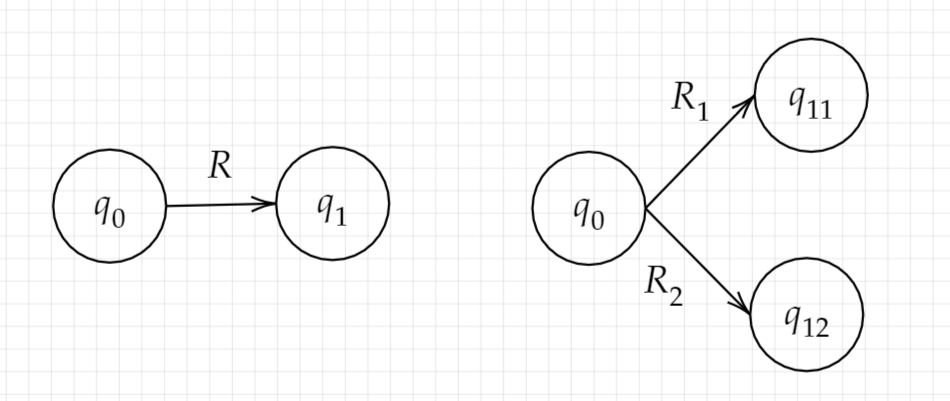1. $R=R_1\circ R_2$
只需将下图的左图转换为右图即可. 如果左图中的$q_1$是接受状态, 则右图中的$q_{12}$是接受状态.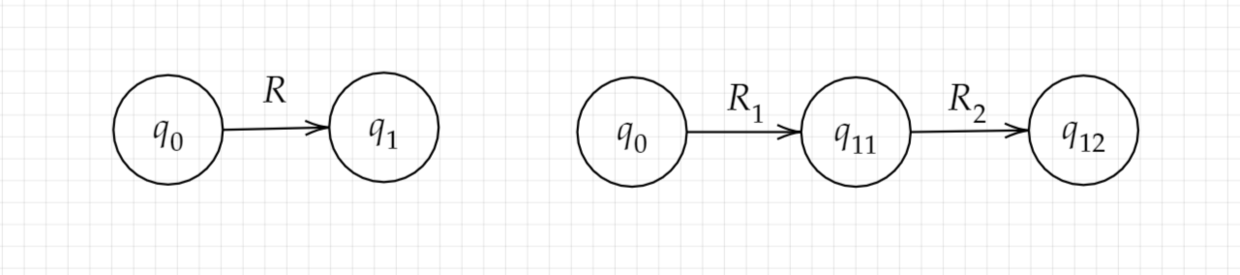1. $R=R_1^\ast$
只需将下图中的作图转换为右图即可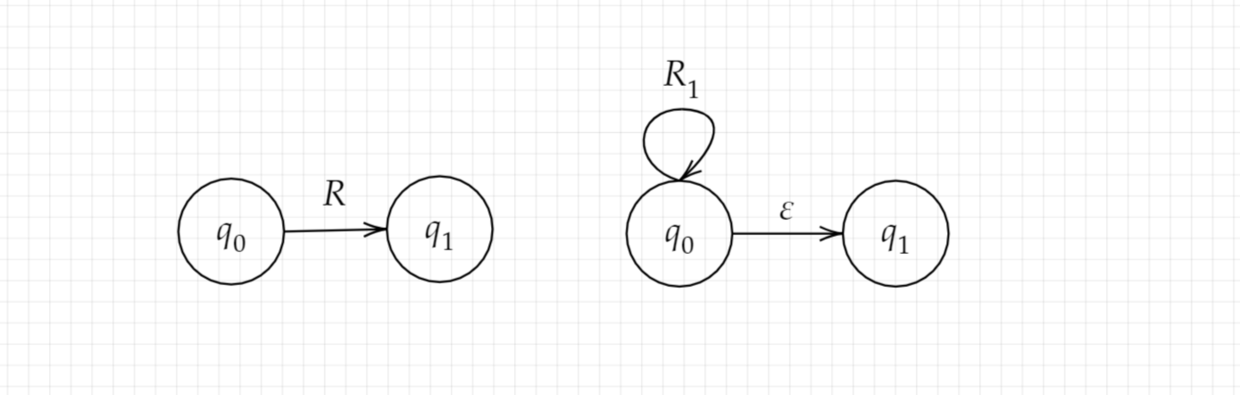# 泵引理

1. ${ \left|xy\right|\leq n}$
2. ${ \left|y\right|\geq 1}$
3. ${ \forall k\geq 0:xy^{k}z\in L}$

# 习题

1. 编写一个判定正则语言的程序$P_1:x\to \{0,1\}$, 程序的输入是一个正则表达式$R$和一个串$x$. 设正则表达式$R$表示的语言为$L$. $P_1(x)=1\iff x\in L$.